This lesson demonstrates the influence of self-steepening effect on short soliton pulses and to compare it with that of the intrapulse Raman scattering.

The layout and global parameters are shown in Figure 1.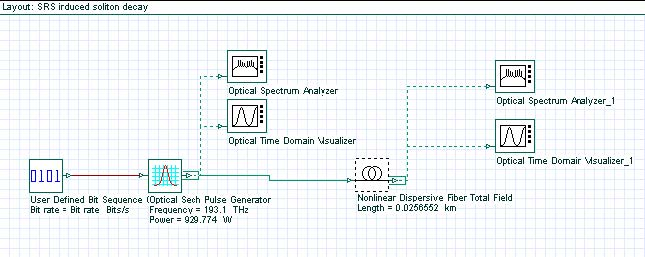The setups for the Bit sequence generator and the sech-pulse generator are shown in figures 2 and 3, respectively.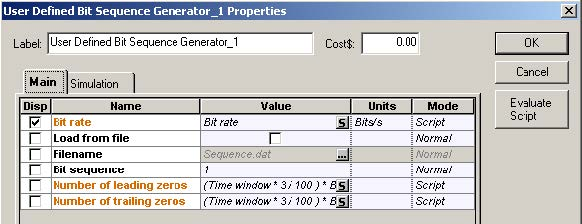Figure 2: Bit sequence generator parameters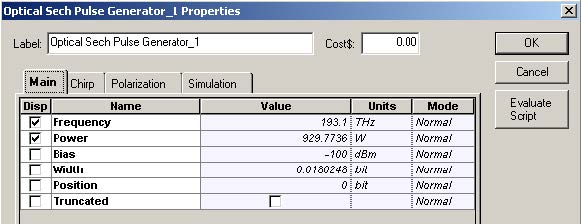Figure 3: Sech-pulse generator parameters

The parameters of the nonlinear dispersive fiber component are shown in figure 4.
The layout simulates N=2 soliton pulse propagation within five soliton periods . The
pulse width (FWHM) is 450.62fs, with the corresponding value of T0 being T0 (TFWHM/1.763) = 255.6fs.

Note that we consider the same pulse and fiber parameters as those considered in the “Decay of higher-order solitons in the presence of intrapulse Raman scattering” except that here we enable the self-steepening effect instead of the intrapulse Raman scattering effect.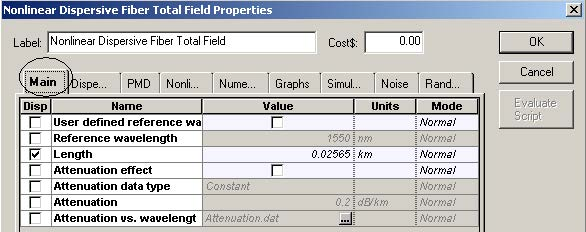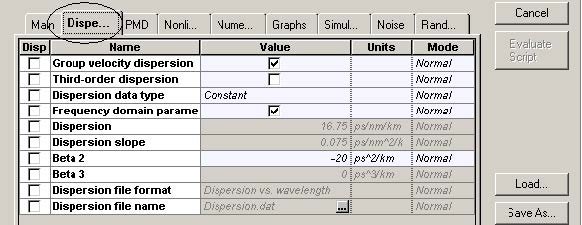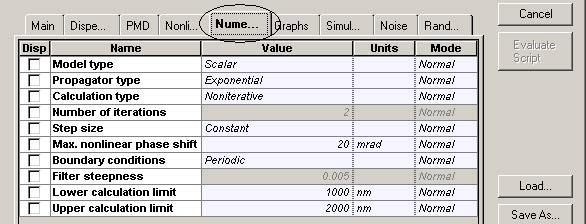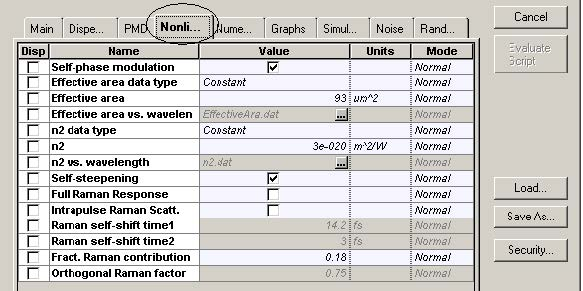Figure 4:  Fiber parameters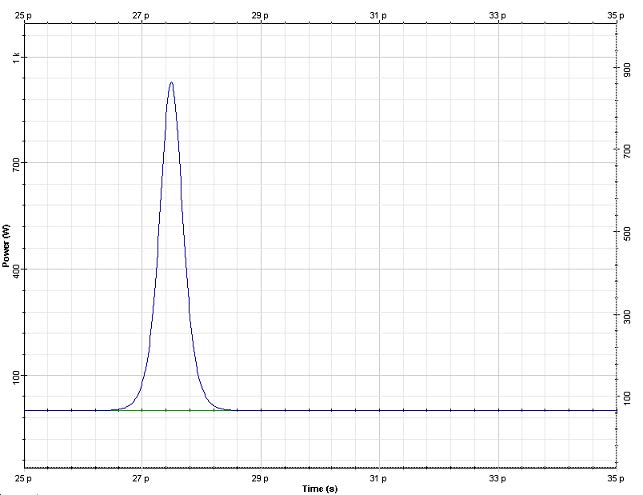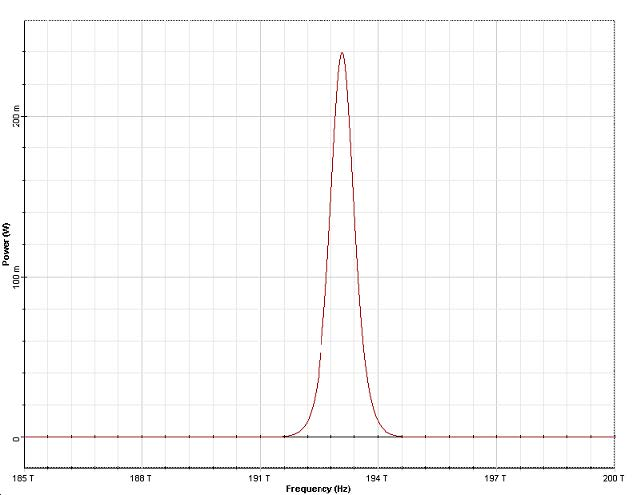Figure 5: Input pulse shape (top) and spectrum (bottom)

Figure 5 shows the input pulse shape and spectrum. The output pulse shape after five and ten soliton periods of propagation are shown in Figure 6. It can be seen that the effect of self-steepening on the higher-order soliton is to split it into its constituents .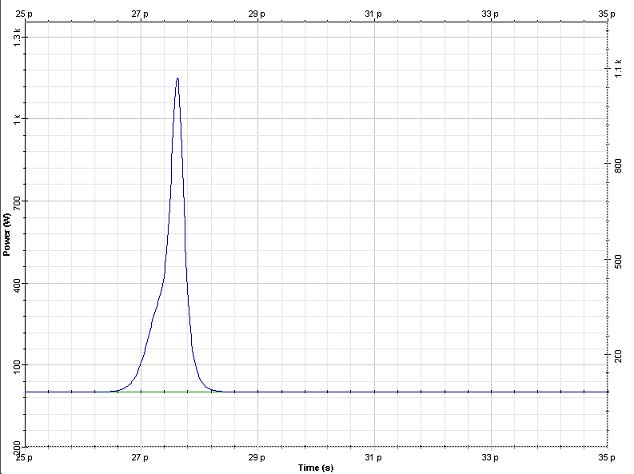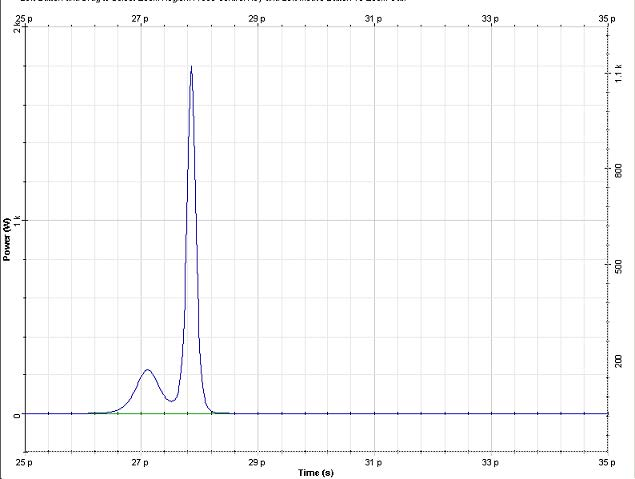Figure 6:  Output pulse shape at five (top) and ten (bottom) soliton periods

Note: Ten soliton periods correspond to 51.3 m fiber length.

While the soliton splitting has occurred within a distance of five soliton periods in the case when the intrapulse Raman scattering acts as a perturbing effect, the splitting is still in its initial stage (for the same input configuration) in the case considered here. This shows that the self-steepening effect is weaker than the stimulated Raman scattering .

Reference:
 G. P. Agrawal Nonlinear Fiber Optics, Academic Press (2001).